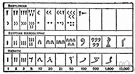# number system

(redirected from Number Systems)
Also found in: Thesaurus, Encyclopedia.

## number system

n.
Any system of naming or representing numbers, as the decimal system or the binary system. Also called numeral system.
ThesaurusAntonymsRelated WordsSynonymsLegend:
 Noun 1number system - any notation for the representation of numbersmathematical notation - a notation used by mathematicianspositional notation, positional representation system - a numeration system in which a real number is represented by an ordered set of characters where the value of a character depends on its positionradix, base - (numeration system) the positive integer that is equivalent to one in the next higher counting place; "10 is the radix of the decimal system"
Translations
References in periodicals archive ?
This book, volume 23 in the series, focuses on the basic number systems of mathematics: natural numbers, integers, rational numbers, real numbers, and complex numbers.
However, we know that it is possible to have number systems based on numbers other than ten.
He introduces the basic number systems of mathematics: natural numbers, integers, rational numbers, real numbers, and complex numbers.
Different number systems were used simultaneously in the Arab world over a long period of time.
These deal with the foundations of number systems in Chapter 1 (Logic), and Chapter 2 (Set Theory).
Chapters cover common number systems and conversions, binary codes, fundamentals of boolean algebra, combinational logic circuits, sequential logic circuits, introduction to ABEL hardware description language, VHDL, Verilog, and Boundary-Scan Architecture, and much more.
At the conclusion of this unit, students had participated in an exploratory approach that introduced them to books from four genres, provided a basic introduction to how libraries are organized using call number systems, and increased their knowledge about an interesting animal.
An impressive, thorough addition to the middle school reference shelf, this summary of time designation and calculations answers the younger YAs' need for data on the beginnings of number systems, clocks, and calendars.
Although I'm interested in math, I've heard and read that the only math left to discover is in the airy range at the top of a mountain of other numbers and number systems.
The dangers of 'seeing English as if it were Language' are well illustrated in Chapter 2, which surveys number systems.
Those seeking a bound volume with the names and properties of any type of metal likely to show up at their facility will be interested in the ninth edition of Metals and Alloys in the Unified Number Systems.

Site: Follow: Share:
Open / Close